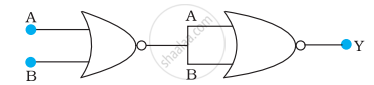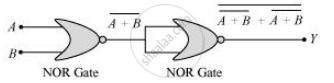# Write the truth table for circuit given in figure below consisting of NOR gates and identify the logic operation (OR, AND, NOT) which this circuit is performing. - Physics

Numerical

Write the truth table for circuit given in figure below consisting of NOR gates and identify the logic operation (OR, AND, NOT) which this circuit is performing.(Hint: A = 0, B = 1 then A and B inputs of second NOR gate will be 0 and hence Y=1. Similarly work out the values of Y for other combinations of A and B. Compare with the truth table of OR, AND, NOT gates and find the correct one.)

#### Solution

A and B are the inputs of the given circuit. The output of the first NOR gate is bar(A +B). It can be observed from the following figure that the inputs of the second NOR gate become the out put of the first one.Hence, the output of the combination is given as:

Y = bar(bar("A+B") + bar("A+B")) = bar(bar("A").bar("B")) + bar(bar"A".bar"B")

= bar(bar"A".bar"B") = bar(bar"A") + bar(bar"B") = "A + B"

The truth table for this operation is given as:

 A B Y (=A + B) 0 0 0 0 1 1 1 0 1 1 1 1

This is the truth table of an OR gate. Hence, this circuit functions as an OR gate.

Concept: Digital Electronics and Logic Gates
Is there an error in this question or solution?

#### APPEARS IN

NCERT Physics Part 1 and 2 Class 12
Chapter 14 Semiconductor Electronics: Materials, Devices and Simple Circuits
Exercise | Q 14.14 | Page 499
NCERT Class 12 Physics Textbook
Chapter 14 Semiconductor Electronics: Materials, Devices and Simple Circuits
Exercise | Q 18 | Page 511

Share